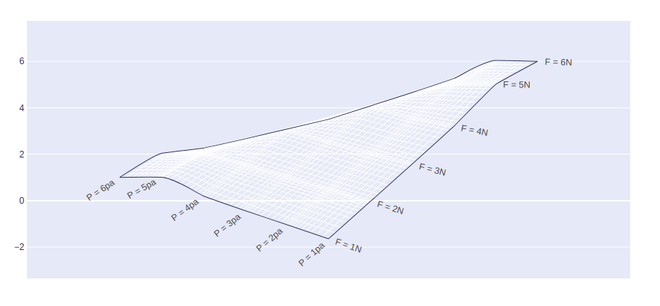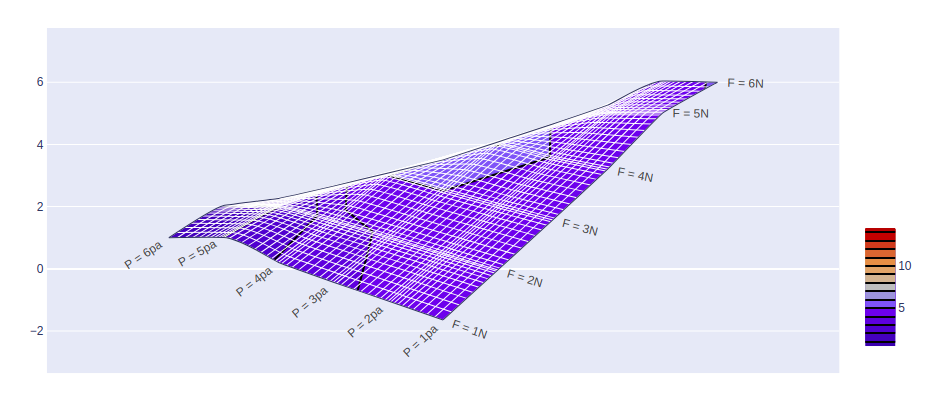# Carpet Contour Plot using Plotly in Python

A Plotly is a Python library that is used to design graphs, especially interactive graphs. It can plot various graphs and charts like histogram, barplot, boxplot, spreadplot, and many more. It is mainly used in data analysis as well as financial analysis. plotly is an interactive visualization library.

## Carpet Contour Plot

A carpet contour plot is a graphical technique for representing a 3-dimensional surface by plotting constant z slices, called contours, on a 2-dimensional format. X and Y attributes are used to set the x and y coordinates. In case the value of x is missing then it will form a cheater plot. A and B attributes should be used to save the values of the parameter.

Syntax: plotly.graph_objects.Contourcarpet( a=None,b=None,xaxis=None, yaxis=None, z=None)

Parameters:

Example:

## Python3

 `import` `plotly.graph_objects as go ` ` `  `fig ``=` `go.Figure(go.Carpet( ` `    ``a``=``[``1``, ``2``, ``3``, ``4``, ``5``, ``6``], ` `    ``b``=``[``6``, ``5``, ``4``, ``3``, ``2``, ``1``], ` `    ``y``=``[``1``, ``2``, ``3``, ``4``, ``5``, ``6``], ` ` `  `    ``aaxis``=``dict``( ` `        ``tickprefix``=``'F = '``, ` `        ``ticksuffix``=``'N'``, ` `        ``smoothing``=``0.2``, ` `        ``minorgridcount``=``10``, ` `    ``), ` `    ``baxis``=``dict``( ` `        ``tickprefix``=``'P = '``, ` `        ``ticksuffix``=``'pa'``, ` `        ``smoothing``=``0.4``, ` `        ``minorgridcount``=``9``, ` `    ``) ` `)) ` ` `  `fig.show() `

Output:In plotly, contours can be added by using the go.Contourcarpet() method of graph_object class. Contours should be added in such a way that the graph body should be in the proper segment.

Example:

## Python3

 `import` `plotly.graph_objects as go ` ` `  `fig ``=` `go.Figure() ` ` `  `fig.add_trace(go.Contourcarpet( ` `    ``a ``=` `[``1``, ``2``, ``3``, ``4``, ``5``, ``6``], ` `    ``b ``=` `[``6``, ``5``, ``4``, ``3``, ``2``, ``1``], ` `    ``z ``=` `[``1``, ``1.96``, ``5``, ``6.1028``, ``4``, ``5.0625``], ` `    ``autocontour ``=` `False``, ` `    ``contours ``=` `dict``( ` `        ``start ``=` `1``, ` `        ``end ``=` `14``, ` `        ``size ``=` `1` `    ``), ` `    ``line ``=` `dict``( ` `        ``width ``=` `2``, ` `        ``smoothing ``=` `0` `    ``), ` `    ``colorbar ``=` `dict``( ` `       ``len` `=` `0.4``, ` `        ``y ``=` `0.25` `    ``) ` `)) ` ` `  ` `  `fig.add_trace(go.Carpet( ` `    ``a``=``[``1``, ``2``, ``3``, ``4``, ``5``, ``6``], ` `    ``b``=``[``6``, ``5``, ``4``, ``3``, ``2``, ``1``], ` `    ``y``=``[``1``, ``2``, ``3``, ``4``, ``5``, ``6``], ` ` `  `    ``aaxis``=``dict``( ` `        ``tickprefix``=``'F = '``, ` `        ``ticksuffix``=``'N'``, ` `        ``smoothing``=``0.2``, ` `        ``minorgridcount``=``10``, ` `    ``), ` `    ``baxis``=``dict``( ` `        ``tickprefix``=``'P = '``, ` `        ``ticksuffix``=``'pa'``, ` `        ``smoothing``=``0.4``, ` `        ``minorgridcount``=``9``, ` `    ``) ` `)) ` ` `  `fig.show() `

Output:Whether you're preparing for your first job interview or aiming to upskill in this ever-evolving tech landscape, GeeksforGeeks Courses are your key to success. We provide top-quality content at affordable prices, all geared towards accelerating your growth in a time-bound manner. Join the millions we've already empowered, and we're here to do the same for you. Don't miss out - check it out now!

Previous
Next